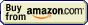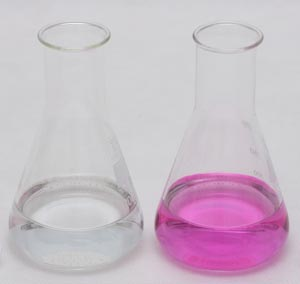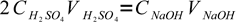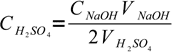# Titrations## Titration » Overview

Titration is a laboratory method of quantitative analysis used to determine unknown concentration of known substance.

Analysis is performed using a burette - kind of laboratory glass made for exact measurement of volume of solution used.

The most popular titrimetric experiment is the determination of an amount of an acid in solution.

Imagine you have a solution of a sulfuric acid of unknown concentration.

Pour an exact measured volume of sulfuric acid (VH2SO4) into a beaker and add few drops of alcoholic phenolphtalein solution. The solution will be colorless, as phenolphtalein becomes pink only in basic solutions (color becomes visible at pH above 8.2).

Now use a burette to slowly add NaOH solution (called titrant) of known molar concentration CNaOH. The will pH slowly go up. Once all of the sulfuric acid becomes neutralized, adding one excess drop of strong base will be enough to rapidly change the pH of the solution and change its color to pink.Color change of phenolphthalein during titration - on the left, colorless solution before end point, on the right - pink solution after end point.

When the color of the solution changes you know that you have neutralized all acid present - you have reached the titration end point. Use the burette scale to read the volume of the titrant used (VNaOH).

We know that one mole of H2SO4 reacts with exactly two moles of NaOH:

2NaOH + H2SO4 → Na2SO4 + 2H2O

Since we know the amount of substance of a given concentration in a given volume of solution is n=C×V, we can write:1

where 2 is a coefficient reflecting stoichiometry of the reaction equation. There is only one unknown in this equation:2

So we have just determined concentration of unknown concentration of sulfuric acid.

Such stoichiometric calculation is the basis of all volumetric analytical methods. They differ when it comes to reagents used and methods of determination of the end point, but the general idea is always the same.

A volumetric determination can be no better than the equipment and technique used in performing it.